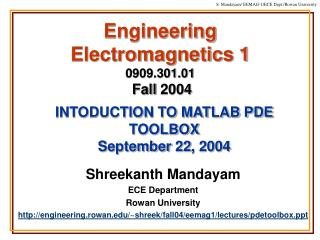# Engineering Electromagnetics 1 0909.301.01 Fall 2004 - PowerPoint PPT PresentationDownload PresentationEngineering Electromagnetics 1 0909.301.01 Fall 2004

Download Presentation## Engineering Electromagnetics 1 0909.301.01 Fall 2004

- - - - - - - - - - - - - - - - - - - - - - - - - - - E N D - - - - - - - - - - - - - - - - - - - - - - - - - - -
##### Presentation Transcript

1. Engineering Electromagnetics 10909.301.01Fall 2004 INTODUCTION TO MATLAB PDE TOOLBOXSeptember 22, 2004 Shreekanth Mandayam ECE Department Rowan University http://engineering.rowan.edu/~shreek/fall04/eemag1/lectures/pdetoolbox.ppt

2. The PDE Toolbox • The Matlab PDE toolbox provides a user-friendly graphical interface for solving 2-D partial differential equations of functions of space and time • Such equations arise in electromagnetics (electrostatics, magnetostatics, quasi-statics), thermal diffusion, structural analysis, etc. • The GUI allows the user to draw 2-D cross-sections of objects, specify sources and boundary conditions and compute the variables (electromagnetic fields, temperatures, stresses, etc.). • A numerical technique called the Finite Element Method (will not be discussed here) is used to solve the pdes.

3. An Example from Electrostatics • The following slides show an example application using the Matlab PDE toolbox. • Problem statement: No Surface Charge V=0 V=10 Laplace’s Equation No Surface Charge

4. Step 1 • Start the toolbox by typing in >> pdetool at the Matlab prompt

5. Step 2 Select Electrostatics Application

6. Step 3 The Draw Menu

7. Step 4 Create Rectangle

8. Step 5 The Boundary Menu

9. Step 6 Display Options

10. Step 7 Select Boundary 4

11. Step 8 Specify Boundary Condition Steps 9 - 10 Select Boundary 1 and specify condition

12. Steps 11 - 14 Specify remaining boundary conditions

13. Step 15 The PDE Mode